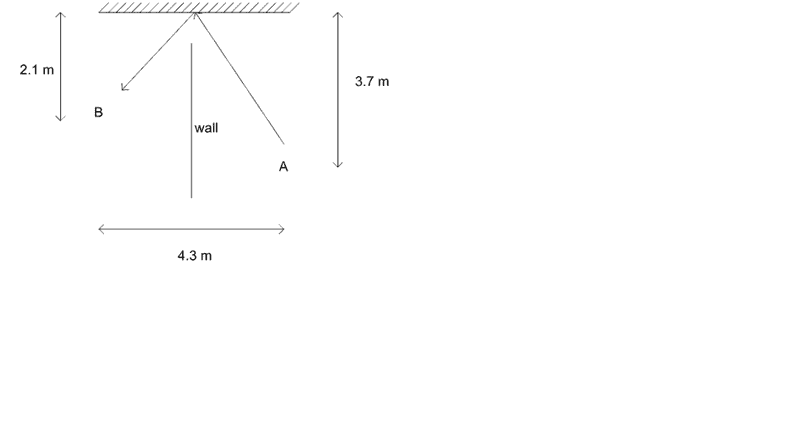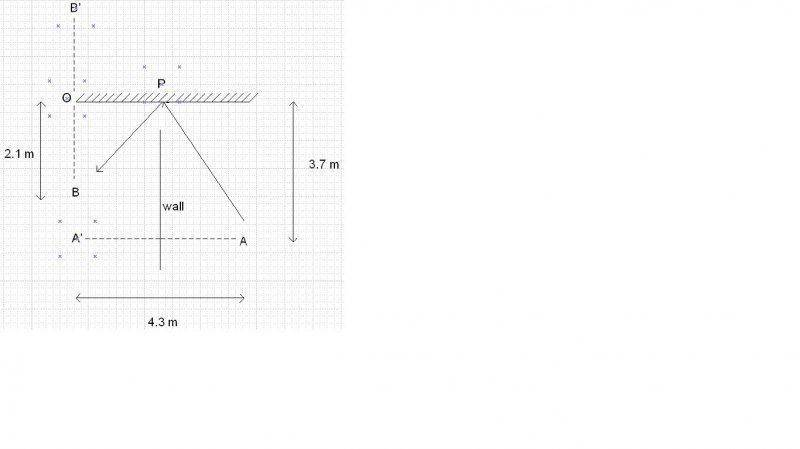# Focal length to get clear image

## Homework StatementHarris stands at point A and wants to take a picture of bird located at point B. Because there is wall, he has to direct his camera to mirror located 3.7 m to the north from his position. What is the focal length of his camera so that he can take a clear picture of the bird?

## Homework Equations

1/f = 1/do + 1/di

f = focal length
do = distance of object
di = distance of image

## The Attempt at a Solution

Harris will see the object as if it appears behind the mirror, let say at position B' (reflection of point B to the mirror), with distance 2.1 m. I try to find object distance (AB') by using Pythagorean theorem (triangle ABB'):

(4.3)2 + (3.7 + 2.1)2 = (BB')2

Is it true the object distance is BB'? If yes, then how to find the distance of image (di)? We have to know di first if we want to calculate f.

Thanks

phinds
Gold Member
2021 Award
(4.3)2 + (3.7 + 2.1)2 = (BB')2
Where did you get that? You need to show your work, not just write down an equation that doesn't seem to mean anything.

Where did you get that? You need to show your work, not just write down an equation that doesn't seem to mean anything.Sorry, I just realized I made mistake.
O is the point on mirror where OB is perpendicular to mirror.
P is point where the light hits the mirror.
OB' is the reflection of OB to the mirror.
A' is point where A is reflected through the wall.

My guess is AB' is the distance of object for the equation. I just realized that AB' may not be a straight line so AA'B' may be not a right - angle triangle at A'. So, I don't know how to find the distance of object and distance of image.

phinds
Gold Member
2021 Award
Hint: angle of incidence = angle of reflection.

Hint: angle of incidence = angle of reflection.

The angle of incidence is tan-1 (2.15 / 3.7) = 30.2o.

Am I correct to say that the object distance is AB'? And what should be the image distance (I mean the distance from what point to what point that will be the image distance)?

Thanks

phinds
Gold Member
2021 Award
Extend the line from the camera to the mirror to a point behind the mirror, then it's just Pythagoras and some simple algebra.

Extend the line from the camera to the mirror to a point behind the mirror, then it's just Pythagoras and some simple algebra.

Ok I got the distance of AB', which is the object distance. To find the focal length of the camera, I have to know the image distance. What is the image distance? Is there special condition for it for getting clear image, such as the image must be formed at normal near point of eye?

Thanks

phinds
Gold Member
2021 Award
What do you think? Do you think you might be able to Google "focal length of a camera" ?

What do you think? Do you think you might be able to Google "focal length of a camera" ?

I tried but I found nothing, or maybe I just didn't understand that I had found the answer through google. What I found mostly the information related to aperture, shutter speed, etc.

I didn't think I had learn that there is special condition to obtain clear image for camera. If the object is really far, the image will be formed on focus point. But for object located at certain position, we adjusted the image distance so that we can obtain clear image. So I don't know how to find the image distance.

Thanks

phinds